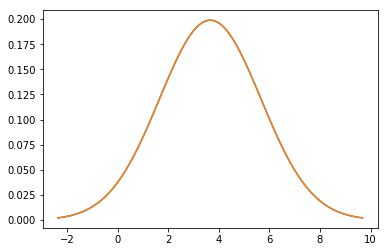Privacy Policy Applying Markov Inequality and Central Limit Theorem on Pomodoro Records to Estimate the Probability to Improve Daily Performance - Andrea Ciufo

# Applying Markov Inequality and Central Limit Theorem on Pomodoro Records to Estimate the Probability to Improve Daily Performance

One day I will improve how to publish a better post from Jupiter on WordPress, all is still work in progress.

The script, that you can find on my GitHub,  will estimate based on my past records the probability to study more Python (or whatever variable are you tracking), in terms of Pomodoro time slots dedicated, based on past records.

Enjoy!

In :

```#THIS IS THE 5th Ver OF THE Clock Work Pomodoro Estimator
#Is still work in progress

# The script is based on the pomodoro technique
#Reads the csv with the past logs recorded and estimates the probability to dedicate more or less pomodoro time slots
#on daily basis on a target activity
#The script based on the past records and the central limit theorem
#with the strong hypothesis that the average daily pomodoro slots follow a normal distribution

#to do: How to plot more figure
#Merging standard deviation and mean from dataframe
#to do:identify the mean problems, specifically understand the value under the denominator

import pandas as pd
import matplotlib.pyplot as plt
import matplotlib.mlab as mlab
import numpy as np
from datetime import datetime, timedelta
import scipy.stats

va='Python'

tvoi='Durata'
#select the number of pomodoros for a selected activity for knowing, based on time series, the probability to improve
improve_pomodoro=8

csvname="logs.csv"
#read the csv with the logged data

dfraw.columns=['Anno', 'Mese', 'Giorno', 'Tempo', 'Durata', 'Avvio (secondi trascorsi)', 'Fine (secondi trascorsi)','Attivit']

#tvoi in this case is the lenght of the single record
#for some reasons are not in the same format
#some records, for a bug, are longer than
#00:00:00
#for this reason we (brutally) clean the data removing all the information longer
#we can consider outliers

#tvoi is a non null object
#we need to convert to string in order to clean the data
dfraw[tvoi] = dfraw[tvoi].astype('str')
dfraw[tvoi]=dfraw[tvoi].str.strip()
#Converting date time to minutes and second removing hours
#they are alway 00 because pomodoro slots last 25 minutes
dfraw[tvoi]=pd.to_datetime(dfraw[tvoi], format='%M:%S')
#Avvio column is expressed in Epoch we can use later as a new index
#useful for resampling

dfraw['IndexDate']=pd.to_datetime(dfraw['Avvio (secondi trascorsi)'], unit='s')
dfraw=dfraw.reset_index().set_index('IndexDate')

#extract all the row contains va word in our case is Python
Python_df=dfraw[dfraw['Attivit'].str.contains(va,na=False)].copy()

Python_df['Date'] = Python_df.apply(lambda row: datetime(row['Anno'], row['Mese'], row['Giorno']), axis=1)

#resample the subset in order to calculate weekly count and then the weekly mean
resample_prova=Python_df.resample('D').count()
#Calculating the std of our dataframe
resample_prova_2=resample_prova.resample('W').mean()

resample_w_std=resample_prova.resample('W').std()
#Converting from a dataframe to a single array
total_std=resample_w_std.std()
total_mean=resample_prova_2.mean()

#To find the probability that the variable has a value LESS than or equal
#of the target "improve pomodoro"is based on the cumulative Density Function

probability_less=scipy.stats.norm.cdf(improve_pomodoro,total_mean,total_std)

print("The probability to dedicate daily less than or equal of %s pomodoro is" %(improve_pomodoro) , probability_less,  '%')

#To find the probability that the variable has a value greater than or
#equal of the "improve pomodoro" is based on the survival function
probability_more=scipy.stats.norm.sf(improve_pomodoro,total_mean,total_std)
print("The probability to dedicate daily more than %s pomodoro is" %(improve_pomodoro) , probability_more , '%' )

#With Markov
print("The probability to dedicate daily more than %s pomodoro based on the Markov Inequality with the average of pomodoro dedicated %s IS" %(improve_pomodoro,total_mean))
print(total_mean/8,'%' )

x = np.linspace(total_mean - 3*total_std,total_mean + 3*total_std, 100)
plt.plot(x,mlab.normpdf(x, total_mean, total_std))
plt.plot(x,mlab.normpdf(x, total_mean, total_std))
plt.show()
```
```The probability to dedicate daily less than or equal of 8 pomodoro is 0.984711072229 %
The probability to dedicate daily more than 8 pomodoro is 0.0152889277713 %
The probability to dedicate daily more than 8 pomodoro based on the Markov Inequality with the average of pomodoro  3.66206896552 dedicated dailyIS
0.45775862069 %
```This site uses Akismet to reduce spam. Learn how your comment data is processed.